# Best-fit line in Linear Regression

### Best Fit Line in Linear Regression

In this blog, let’s understand the Simple Linear Regression and how to find the best fit line in Linear Regression Line.

Simple Linear Regression is the line where the dependent/output variable is based on only one independent variable.

For example:

y=β0+β1x

In the above example, the dependent variable(y) is dependent upon only one independent variable(x).

β0 and β1 are coefficients.

Let’s take a simple example where a manager of an online shopping company wants to predict the sales number in the current month based on money spent on advertising. To predict, the manager needs previous moths data about the number of sales and money spent.

 ad_spending Sales (In thousands) 1000 139 1500 155 1800 160 2000 210 800 120 1200 150 1400 145 1700 164 1100 125

Upon plotting, we can observe the trend. The sales in the online platform are increasing based on the amount spent on advertising.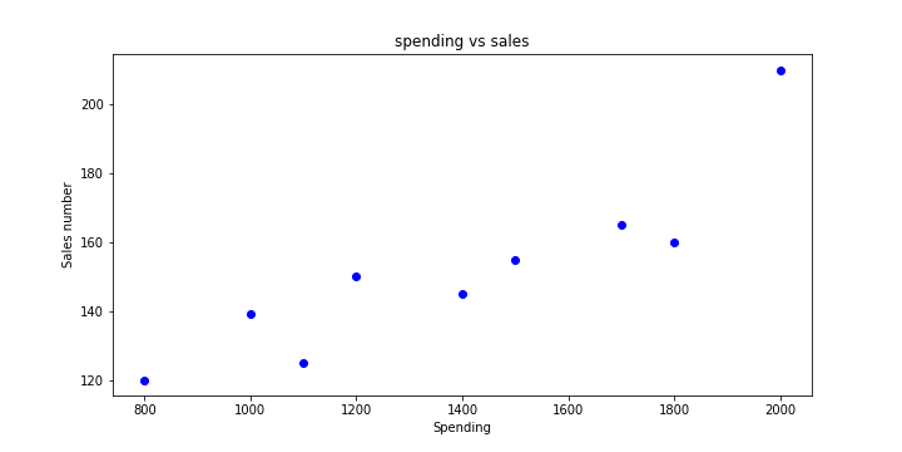Clearly, we cannot put a linear line on each data point, however, we will try to fit a line which is the best fit for these data points.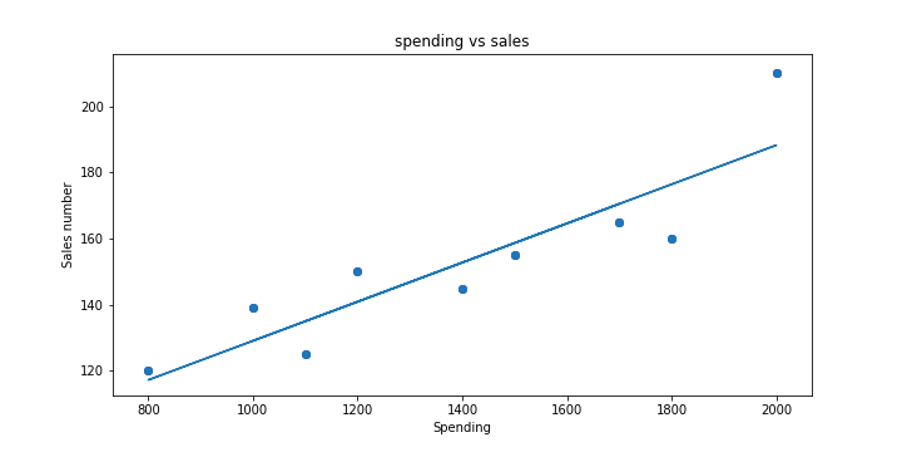Now we can predict the sales volume if the advertising budget is 1600 USD. The sales number will be around 166.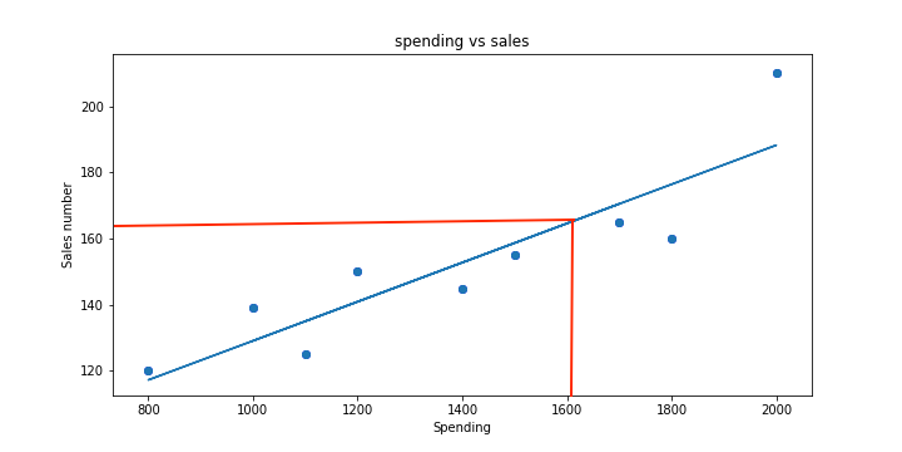##### Best-fit line in Linear Regression:

There could be multiple straight lines which can fit these data point. So now the important question is, which is the best-fit line and how to find it. Before finding the best-fit line let’s understand a few other important concepts.

Residual:

Residual is basically the difference of predicted value from actual values. In another term, we can say the error between the predicted value and actual values. If you observe the below diagram, you will notice that each point is not accurately classified. So, at each data point, the best fit line gives the predicted value.

So, we can write the residue as below: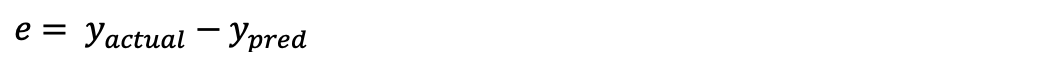The residue should be calculated for each data point. We can rewrite the error term as below.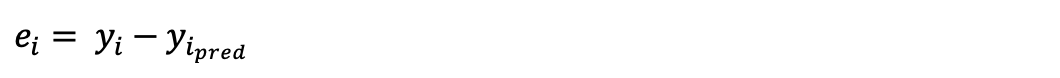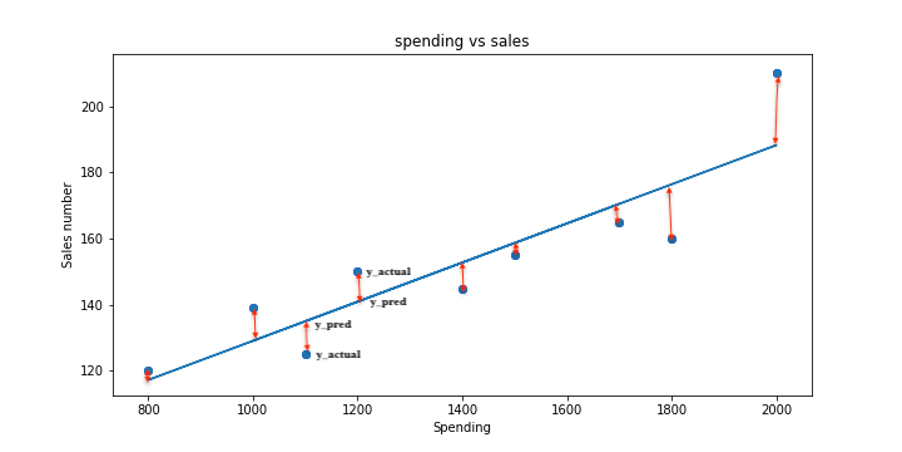To find the best fit line we have to calculate the residual sum of squares.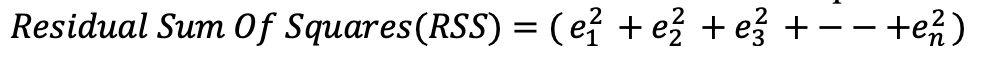This can be rewritten as below: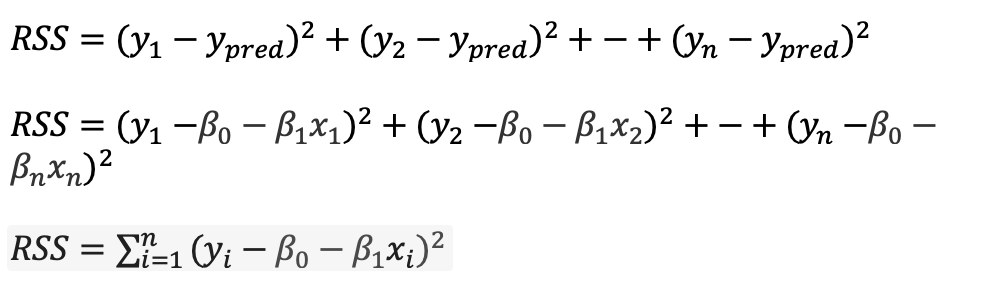To identify the best fit line in linear regression we have to find the line which has minimum Residual Sum of Square (RSS). To find minimize RSS function to find optimal β0 and  β1.

Before understanding how to find β0 and  β1, let’s understand the concept of Cost Function in the next chapter. Follow this link to learn more about Linear Regression.